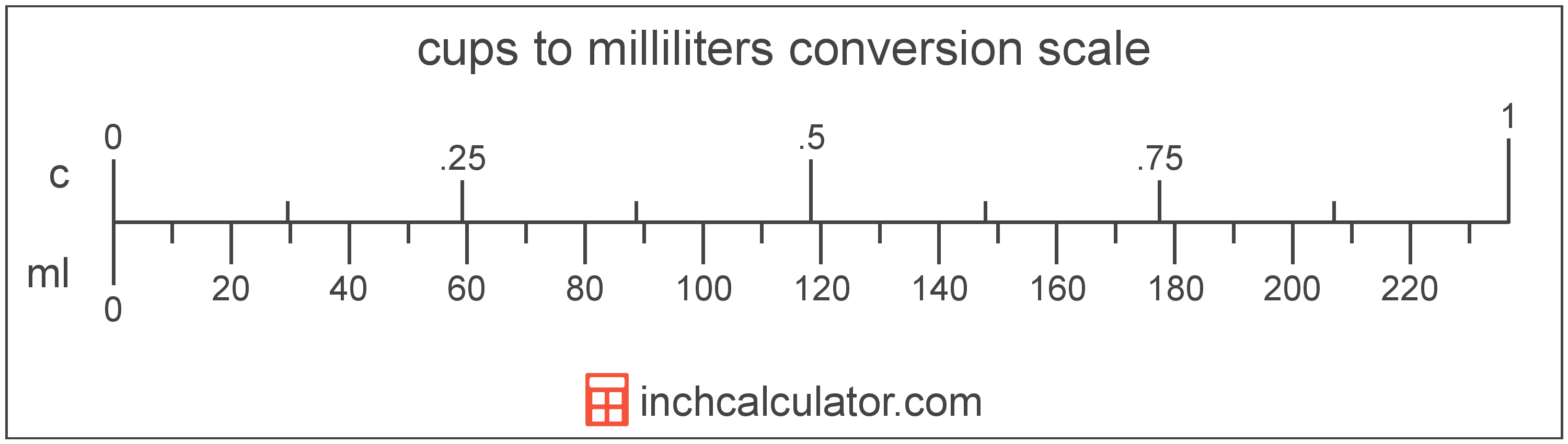# Cups to Milliliters Converter

Enter the volume in cups below to get the value converted to milliliters.

Results in Milliliters:1 c = 236.588236 mL

Do you want to convert milliliters to cups?

## How to Convert Cups to Milliliters

To convert a measurement in cups to a measurement in milliliters, multiply the volume by the following conversion ratio: 236.588236 milliliters/cup.

Since one cup is equal to 236.588236 milliliters, you can use this simple formula to convert:

milliliters = cups × 236.588236

The volume in milliliters is equal to the volume in cups multiplied by 236.588236.

For example, here's how to convert 5 cups to milliliters using the formula above.
milliliters = (5 c × 236.588236) = 1,182.941182 mL### How Many Milliliters Are in a Cup?

There are 236.588236 milliliters in a cup, which is why we use this value in the formula above.

1 c = 236.588236 mL

## What is a Cup?

The cup is a unit of volume equal to 16 tablespoons or 8 fluid ounces. The cup should not be confused with the metric cup or the teacup, which are different units of volume. One cup is equal to 236.588 milliliters, but in nutrition labeling, one cup is rounded to 240 milliliters.

The cup is a US customary unit of volume. Cups can be abbreviated as c, and are also sometimes abbreviated as C. For example, 1 cup can be written as 1 c or 1 C.

## What is a Milliliter?

The milliliter is a unit of volume equal to 1 cubic centimeter, 1/1,000 of a liter, or about 0.061 cubic inches.

The milliliter is an SI unit of volume in the metric system. In the metric system, "milli" is the prefix for thousandths, or 10-3. A milliliter is sometimes also referred to as a millilitre. Milliliters can be abbreviated as mL, and are also sometimes abbreviated as ml or mℓ. For example, 1 milliliter can be written as 1 mL, 1 ml, or 1 mℓ.

Since one milliliter is equivalent to one cubic centimeter, milliliters are sometimes expressed using the abbreviation for a cubic centimeter (cc) for things such as medical dosages or engine displacements.

## Cup to Milliliter Conversion Table

Table showing various cup measurements converted to milliliters.
Cups Milliliters
1 c 236.59 mL
2 c 473.18 mL
3 c 709.76 mL
4 c 946.35 mL
5 c 1,183 mL
6 c 1,420 mL
7 c 1,656 mL
8 c 1,893 mL
9 c 2,129 mL
10 c 2,366 mL
11 c 2,602 mL
12 c 2,839 mL
13 c 3,076 mL
14 c 3,312 mL
15 c 3,549 mL
16 c 3,785 mL
17 c 4,022 mL
18 c 4,259 mL
19 c 4,495 mL
20 c 4,732 mL
21 c 4,968 mL
22 c 5,205 mL
23 c 5,442 mL
24 c 5,678 mL
25 c 5,915 mL
26 c 6,151 mL
27 c 6,388 mL
28 c 6,624 mL
29 c 6,861 mL
30 c 7,098 mL
31 c 7,334 mL
32 c 7,571 mL
33 c 7,807 mL
34 c 8,044 mL
35 c 8,281 mL
36 c 8,517 mL
37 c 8,754 mL
38 c 8,990 mL
39 c 9,227 mL
40 c 9,464 mL

## References

1. U.S. Food & Drug Administration, Guidance for Industry: Guidelines for Determining Metric Equivalents of Household Measures, https://www.fda.gov/regulatory-information/search-fda-guidance-documents/guidance-industry-guidelines-determining-metric-equivalents-household-measures
2. National Institute of Standards and Technology, Specifications, Tolerances, and Other Technical Requirements for Weighing and Measuring Devices, Handbook 44 - 2019 Edition, https://nvlpubs.nist.gov/nistpubs/hb/2019/NIST.HB.44-2019.pdf